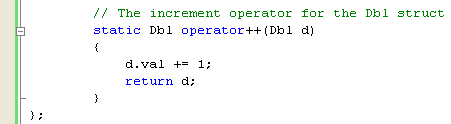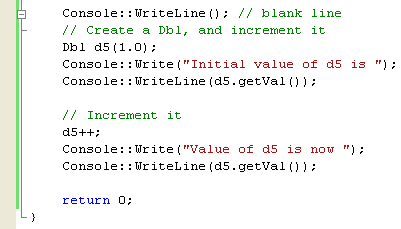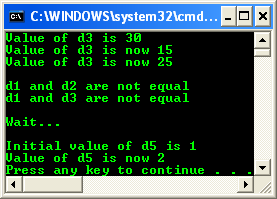5. # Guidelines for Providing Overloaded Operators

Implementing Increment and Decrement

As a final example, the exercise in this section shows you how to overload the increment and decrement operators (++ and --). The built-in ++ and -- operators are used to increment and decrement the values of numeric variables. You can overload them for your own numeric types, but you can also overload these operators to provide other functionality. For example, suppose you had a Date type that holds day, month, and year values; you could implement ++ and -- to add or subtract one day from the current date, adjusting the month and year as appropriate.

The following exercise shows you how to implement the ++ operator for the Dbl type that you’ve been working with in this module.

1.        Using the same project as in the previous exercises, find the Equals function in your code and add the following code immediately after it:

// The increment operator for the Dbl struct

static Dbl operator++(Dbl d)

{

d.val += 1;

return d;

}The ++ operator is implemented by the operator++ function; the -- operator is implemented by operator--. The single argument represents the value to be incremented, so 1 gets added to its value and then a copy is returned.

2.        Use the operator in code like this:

Console::WriteLine(); // blank line

// Create a Dbl, and increment it

Dbl d5(1.0);

Console::Write("Initial value of d5 is ");

Console::WriteLine(d5.getVal());

// Increment it

d5++;

Console::Write("Value of d5 is now ");

Console::WriteLine(d5.getVal());3.        Build and run your program. The following output should be expected.The answer 2 should print out, representing the new value of d5. Note that even though the function returns a Dbl, the return value isn’t being used in this example and you’re executing the function only to get its side effect of incrementing the value. Once you’ve implemented the increment operator, you’ll find it simple to implement decrement as well. There are two forms of the increment and decrement operators: the prefix form that goes before the operand (++x) and the postfix form that follows the operand (x++). When overloading managed types, it isn’t possible to distinguish between prefix and postfix operators, so the same operator++ or operator-- function is called for both. In traditional C++ operator overloading, you can overload both prefix and postfix versions separately.

You can overload operators for reference types in the same way that you do for value types, but you need to be aware of the issues detailed in the following section.

Implementing Overloaded Operators for Reference Types

You already know that reference types are accessed using pointers, which means that the arguments to operator functions for reference types always have to be pointers. So, if you were to implement operator++ for a reference type, you would have to code it something like this:

// The addition operator for a reference type

static MyRef^ operator++(MyRef^ pLhs, MyRef^ pRhs)

{

MyRef^ result = gcnew MyRef(pLhs->val + pRhs->val);

return result;

}

Calling Overloaded Operators for Reference Types

You can’t call overloaded operators implicitly for reference types, so you have to call the overload function directly, like this:

// This doesn’t work

MyRef^ r3 = one + two;

// This does

MyRef^ r3 = MyRef::operator++(one, two);

The most important guideline is: Overloaded operators must make intuitive sense for a class. For instance, if you have a String class, using + to concatenate the Strings is pretty intuitive. You might get some agreement that - , as in s2- s1, would mean “look for s1 within s2, and if you find it, remove it.” But what could the * operator mean when applied to two Strings? There’s no obvious meaning, and you’re only going to confuse people if you provide it. So make sure that the operators you provide for your types are the ones that people expect to find. The second guideline is: Operator usage must be consistent. In other words, if you overload ==, make sure you overload != as well. The same goes for < and >, ++ and --, and so on. The C# compiler enforces consistent overloading, and it won’t let you overload one of a pair of operators without also implementing the other. In C++, however, it is up to you to be consistent.

The third guideline is: Don’t overload obscure operators or ones that change the semantics of the language. Operators such as the comma are obscure, and few people know how they work, so it isn’t a good idea to overload them. Other operators, such as the logical AND and OR operators (&& and ||), can cause problems. In earlier module, you learned about the if statement and how expressions joined by && and || are only evaluated if necessary. As a result, some expressions in an if statement might never be evaluated. If you overload the AND and OR operators, the whole of the expression will have to be evaluated, which changes the way the if works.

A complete code for the practice with some cosmetic changes for this module is given below.

// Compile with /clr

#include "stdafx.h"

using namespace System;

// The Dbl struct definition

value struct Dbl

{

double val;

// creation or assignment time

double hr, min, sec;

public:

Dbl(double v)

{

val = v;

// Create new date and time fields

DateTime dt = DateTime::Now;

hr = dt.Hour;

min = dt.Minute;

sec = dt.Second;

}

double getVal() { return val; }

// The addition operator for the Dbl struct

// lhs - left hand side, rhs - right hand side

static Dbl operator+(Dbl lhs, Dbl rhs)

{

Dbl result(lhs.val + rhs.val);

return result;

}

// Adding a Dbl and an int

static Dbl operator+(Dbl lhs, int rhs)

{

Dbl result(lhs.val + rhs);

return result;

}

// Adding an int and a Dbl

static Dbl operator+(int lhs, Dbl rhs)

{

Dbl result(lhs + rhs.val);

return result;

}

// The equality operator for the Dbl struct

static bool operator==(Dbl lhs, Dbl rhs)

{

return lhs.val == rhs.val;

}

// The inequality operator for the Dbl struct

static bool operator!=(Dbl lhs, Dbl rhs)

{

return !(lhs == rhs);   // calls op_Equality()

}

// The equality operator for the Dbl struct

virtual bool Equals(Object^ pOther) override

{

// cast pOther to Dbl

Dbl^ s = dynamic_cast<Dbl^>(pOther);

// test whether it is the null pointer

if (s == nullptr)

return false;

else

return s->val == val;

}

// The assignment operator for the Dbl struct

static Dbl op_Assign(Dbl& lhs, const Dbl& rhs)

{

// Copy over the value

lhs.val = rhs.val;

// Create new date and time fields

DateTime dt = DateTime::Now;

lhs.hr = dt.Hour;

lhs.min = dt.Minute;

lhs.sec = dt.Second;

return lhs;

}

// The increment operator for the Dbl struct

static Dbl operator++(Dbl d)

{

d.val += 1;

return d;

}

};

int main()

{

Dbl d1(10.0);

Dbl d2(20.0);

Dbl d3(0.0);

Console::WriteLine("d1 is {0}", d1.getVal());

Console::WriteLine("d2 is {0}", d2.getVal());

Console::WriteLine("d3 is {0}", d3.getVal());

Console::WriteLine(); // blank line

d3 = d1 + d2;

Console::Write("Value of d3 = d1 + d2 is ");

Console::WriteLine(d3.getVal());

// Add a Dbl and an int

d3 = d1 + 5;

Console::Write("Value of d3 = d1 + 5 now ");

Console::WriteLine(d3.getVal());

// Add an int and a Dbl

d3 = 5 + d2;

Console::Write("Value of d3 = 5 + d2 now ");

Console::WriteLine(d3.getVal());

Console::WriteLine(); // blank line

if (d1 == d2)

Console::WriteLine("d1 and d2 are equal");

else

Console::WriteLine("d1 and d2 are not equal");

if (d1.Equals((Object^)d3))

Console::WriteLine("d1 and d3 are equal");

else

Console::WriteLine("d1 and d3 are not equal");

Console::WriteLine(); // blank line

// Create two objects

Dbl one(0.0);

Dbl two(10.0);

// Sleep for five seconds

Console::WriteLine("Wait... ");

// Do an assignment

one = two;

Console::WriteLine(); // blank line

// Create a Dbl, and increment it

Dbl d5(1.0);

Console::Write("Initial value of d5 is ");

Console::WriteLine(d5.getVal());

// Increment it

d5++;

Console::Write("Value of d5 is now ");

Console::WriteLine(d5.getVal());

return 0;

}

Output:Well, for the managed type of the .NET, you can replace the double with Double and rebuild and re-run your code.